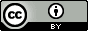## Sunday, December 16, 2012

### Conformational and rotational entropy and point group symmetry

The point group affects the free energy
The use of point group symmetry in quantum chemical calculations can speed up calculations significantly, but it is often difficult to input symmetric coordinates correctly so many people opt to run calculations without symmetry (i.e. in $C_1$ symmetry) anyway.  However, the lack of symmetry changes the rotational entropy and, hence, the free energy you compute. So if you have symmetric molecules but choose to run in $C_1$ symmetry you must correct the entropies and free energies.

The rotational entropy is given by$$S_{rot}=R\ln\left(\frac{8\pi^2}{\sigma}\left(\frac{2\pi ekT}{h^2}\right)^{3/2}\sqrt{I_1I_2I_3}\right)$$ $\sigma$ is called the symmetry number and depends on the point group: for example, $\sigma=1$ for $C_1$ and $C_s$, $\sigma=n$ for $C_{nv}$ and $C_{nh}$, $\sigma= 2n$ for $D_{nh}$ and $D_{nd}$, and $\sigma= 12$ or $T_d$.  So the rotational entropy calculated with and without symmetry will differ by $R\ln(\sigma)$:$$S_{rot}=S_{rot}^{C_1}-R\ln(\sigma)$$and similarly for the free energy$$G^\circ=G^{\circ,C_1}+RT\ln(\sigma)$$
An example: $H_2O+Cl^-\rightleftharpoons HOH\cdots Cl^-$
The free energy change for this reaction is$$\Delta G^\circ=\Delta G^{\circ,C_1}+RT\ln\left(\frac{\sigma_{HOH\cdots Cl^-(C_s)}}{\sigma_{H_2O(C_{2v})}\sigma_{Cl^-(C_1)}}\right)\\ \Delta G^\circ=\Delta G^{\circ,C_1}+RT\ln\left(\frac{1}{2\times 1}\right)=\Delta G^{\circ,C_1}-RT\ln (2)$$The corresponding equilibrium constants are$$K=e^{-\Delta G^\circ/RT}=2K^{C_1}$$So, $\sigma$'s accounts for the fact that, because of the symmetry of water, there are two ways of making $HOH\cdots Cl^-$and another way to view $R\ln(2)$ is that it is the conformational entropy of the complex.

A test: $H_2O+NH_3\rightleftharpoons HOH\cdots NH_3$
For the above equilibrium what is $X$ in$$\Delta G^\circ=\Delta G^{\circ,C_1}-RT\ln (X)$$

An exception: $2H_2O\rightleftharpoons HOH\cdots OH_2$
Based on the rules outlined so far one would expect the free energy change for this equilibrium to be$$\Delta G^\circ=\Delta G^{\circ,C_1}-RT\ln (4)$$The factor of four accounts for the fact that there are four ways of making $HO^AH\cdots O^BH_2$. However, since the water molecules are identical there are actually four additional water dimer possibilities for  $HO^BH\cdots O^AH_2$, so$$\Delta G^\circ=\Delta G^{\circ,C_1}-RT\ln (8)$$In general, for $A+A\rightleftharpoons Product$ reactions the symmetry number for $A+A$ is $2\sigma_A^2$rather than $\sigma_A^2$

All these considerations also apply to activation free energies and rate constants as outlined in this excellent paper by Fernández-Ramos et al., which inspired this post.  See also this excellent paper by Gilson and Irikura.Anonymous said...

Hi!
Thanks for this awesome post.
I'm curious what is your take on the continuous symmetry measures (David Avnir, Hebrew University) and their impact on free energy/entropy estimates. They don't seem to be used commonly to correct for such values. any thoughts?
thanks!

Jan Jensen said...

Hi

Never heard of it. Do you have some specific papers in mind?Anonymous said...

Hi,
here is one of the original references:
http://pubs.acs.org/doi/abs/10.1021/ja00046a033

And here is its use in rotational entropy:
http://www.ncbi.nlm.nih.gov/pubmed/12670261

Best,Anonymous said...

Very nice these posts on thermodynamics. Please continue.

Good point, continues simmetry is a very important concept to understand experimental entropic results, but dificult to calculate. A molecule on its most stable conformation is assimetric (for example, biphenyl has a small dihedral), but the results are best understood when considering that is fully simmetric. This is not exactly what is argued by Avnir, but is closer than considering the molecule assimetric.

T.

Jan Jensen said...

T., thanks!

Jan Jensen said...

Unknown said...

How does one calculate the entropy change for a dehydration reaction in aqueous solution? It seems like neither PCM or COSMO calculates the free-energy for this reaction correctly. It should include an "entropy of liberation" which appears to be neglected in most standard packages.

Jan Jensen said...

Not sure if this answers your question but methods like PCM and COSMO are parameterized to reproduce experimental solvation free energies.

It is not obvious how to separate the COSMO and PCM solvation energies into enthalpy and entropy entropy contributions.

Gilson had a go at it in this paper, but I haven't read it closely enough to understand it.
http://dx.doi.org/dx.doi.org/10.1021/ja109904u

The COSMO-RS method can compute enthalpies of solvation.

Hope this helps